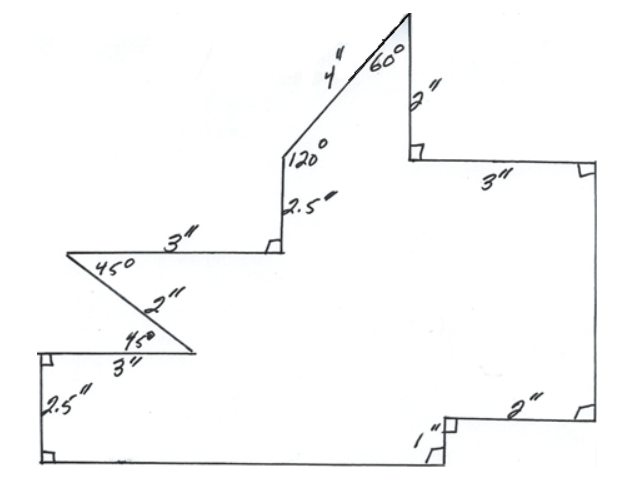# Drawing

So it is time to draw using these two tools!!!!!!

1. Let’s start with a line segment. Draw a 7 inch line segment.
2. Now let’s connect a few segments. Draw a square with a side of 6 inches. Remember that the angles are 90 degrees.
3. Now draw a 30 degree angle.

Now for a tougher one. Draw a rhombus with a side of 5 inches. Make this rhombus have a 50 degree angle. Remember, all sides have the same length.

1. OK….let’s draw an equilateral triangle, with a side of 7 inches. Start with a 7 inch segment.
2. Put a 60 degree on each end of this segment.
3. Extend each side until they meet. This is an equilateral triangle.

Last figure! Let’s draw an isosceles trapezoid. Remember the non-parallel sides are the same length. Make the base 6 inches, and the non-parallel sides 5 inches, and base angles of 70 degrees. So start with the long base of 6 inches. On each end of this put a 70 degrees. Measure 5 inches on each segment, and mark the point. Connect the two points and that’s the trapezoid.

Now accurately draw the shape below. (Your measurements should be accurate. This does not accurately show 4 inches, 2 inches, etc.)From Georgia Virtual Learning

http://cms.gavirtualschool.org/Shared/Math/MSMath7/Drawings/Math7BRulerAndProtractor_Shared/index.html